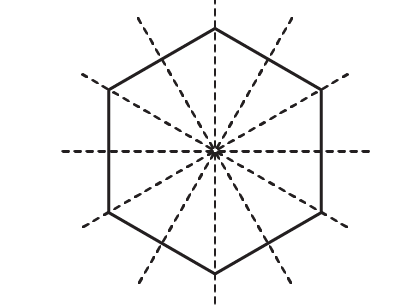# RD Sharma Solutions for Class 6 Maths Chapter 17: Symmetry Exercise 17.3

Here, we get to know about the important figures which contain more than two lines of symmetry. The problems provided help us to understand the symmetry of figures like line, angle, isosceles triangles, equilateral triangles, parallelogram, rhombus, rectangle etc. The solutions are available for free download to help students score well in the exam. In order to solve exercise wise problems based on textbook of current CBSE syllabus, students can refer to RD Sharma Solutions Class 6 Maths Chapter 17 Symmetry Exercise 17.3 PDF which are provided here.

## RD Sharma Solutions for Class 6 Maths Chapter 17: Symmetry Exercise 17.3 Download PDF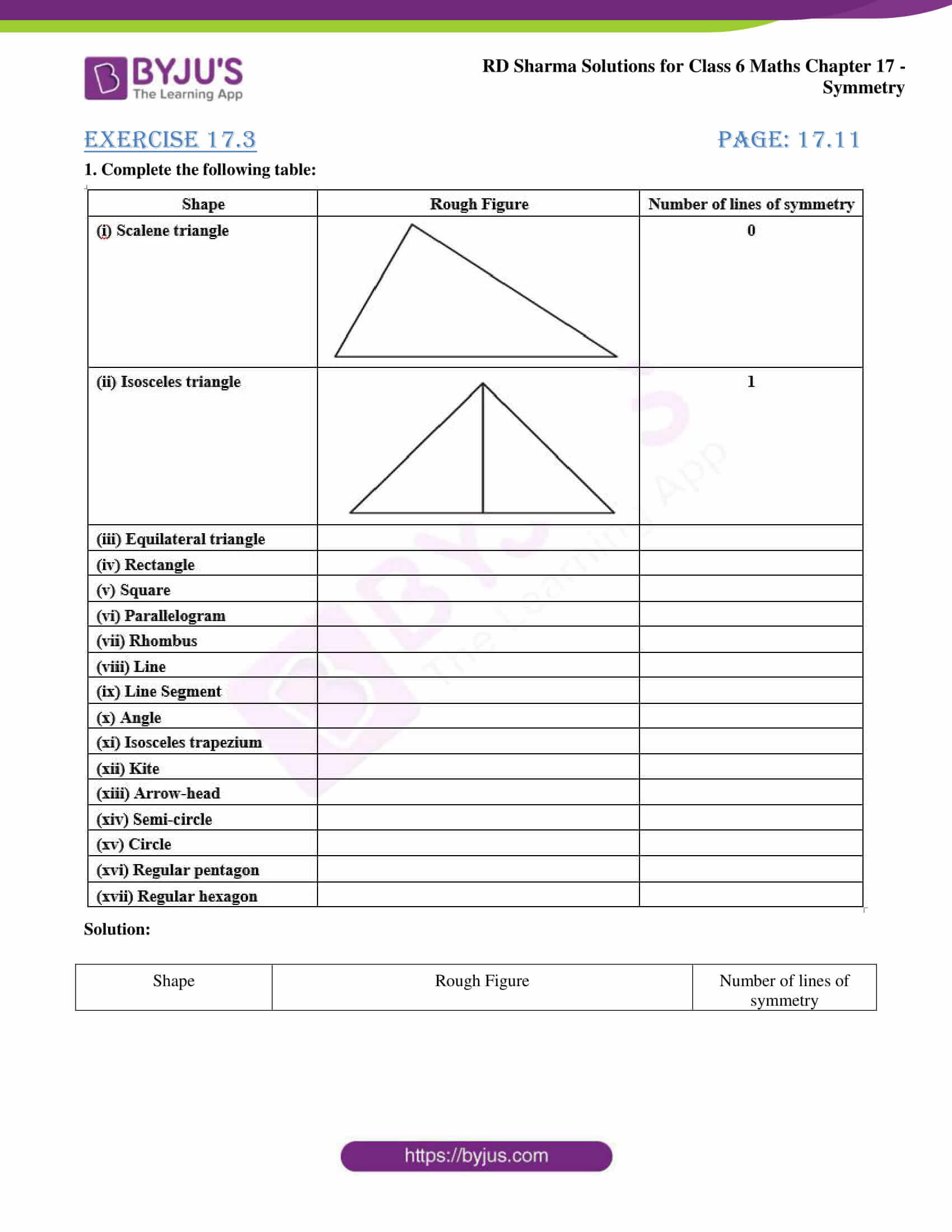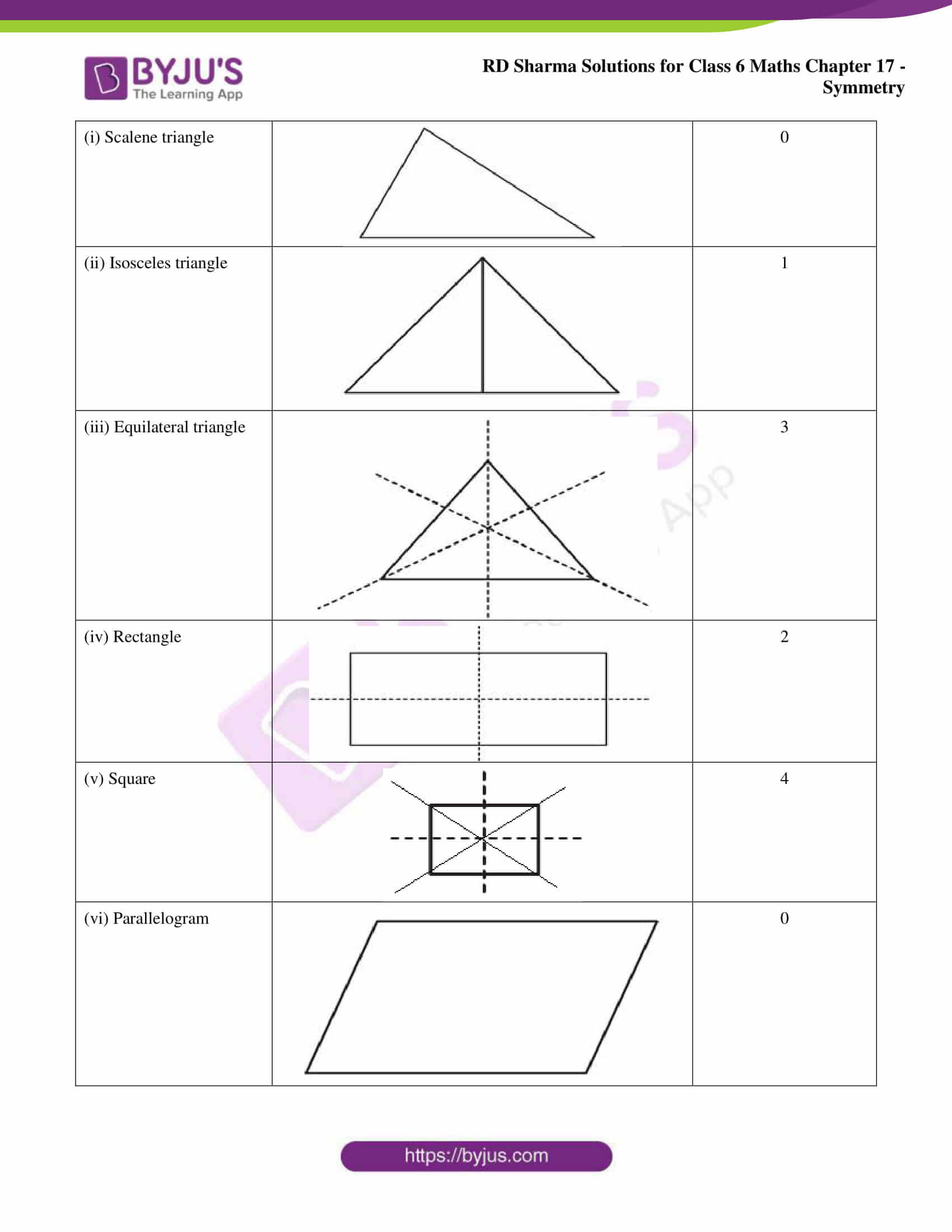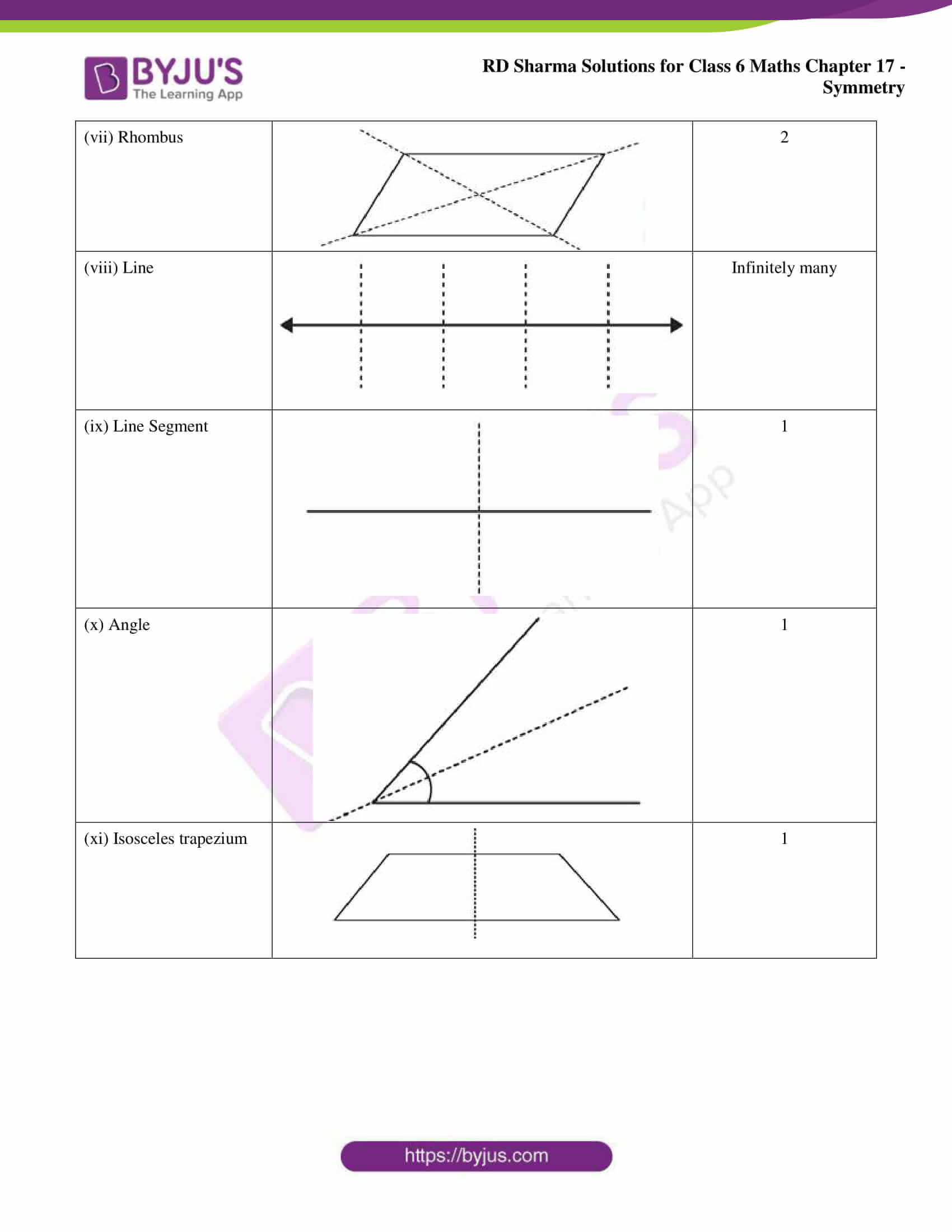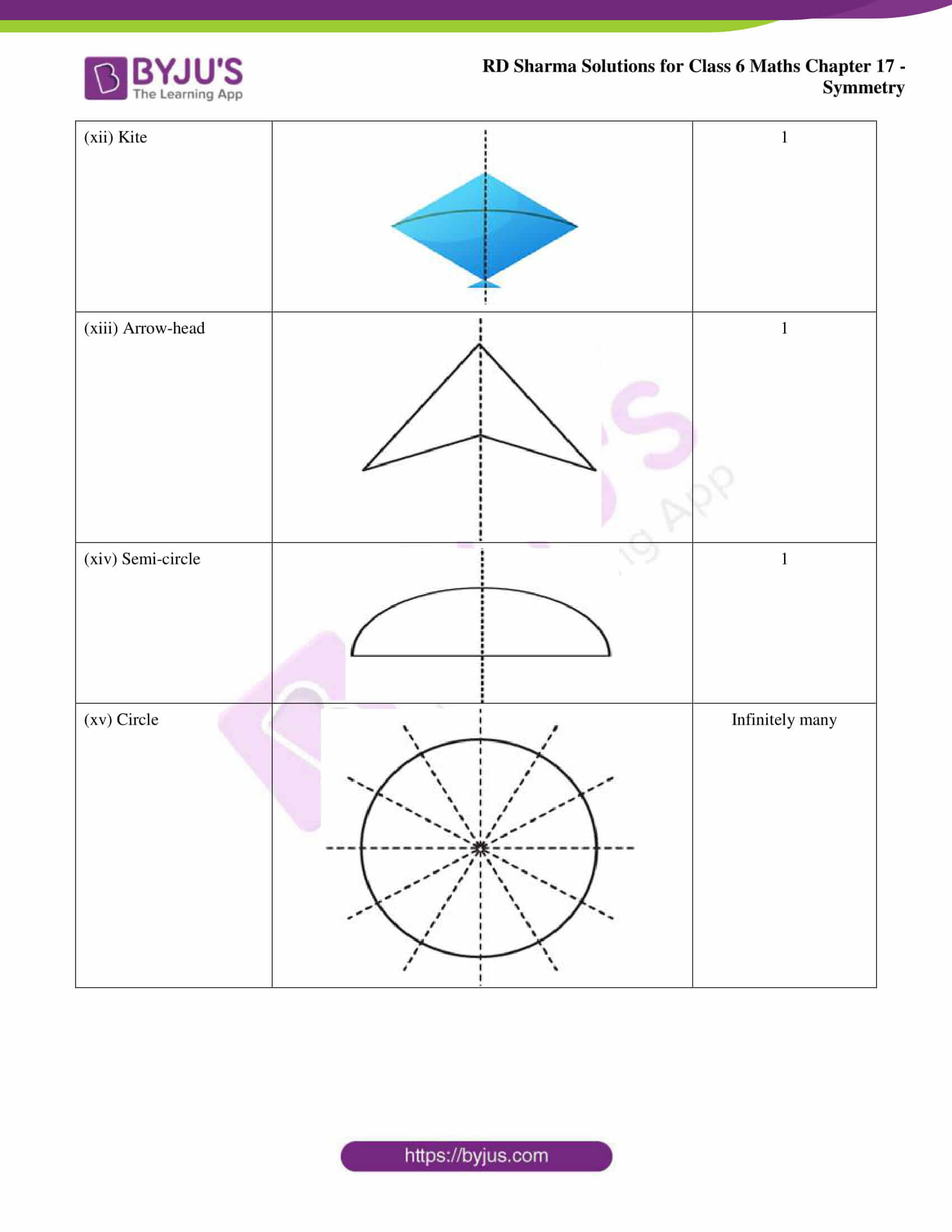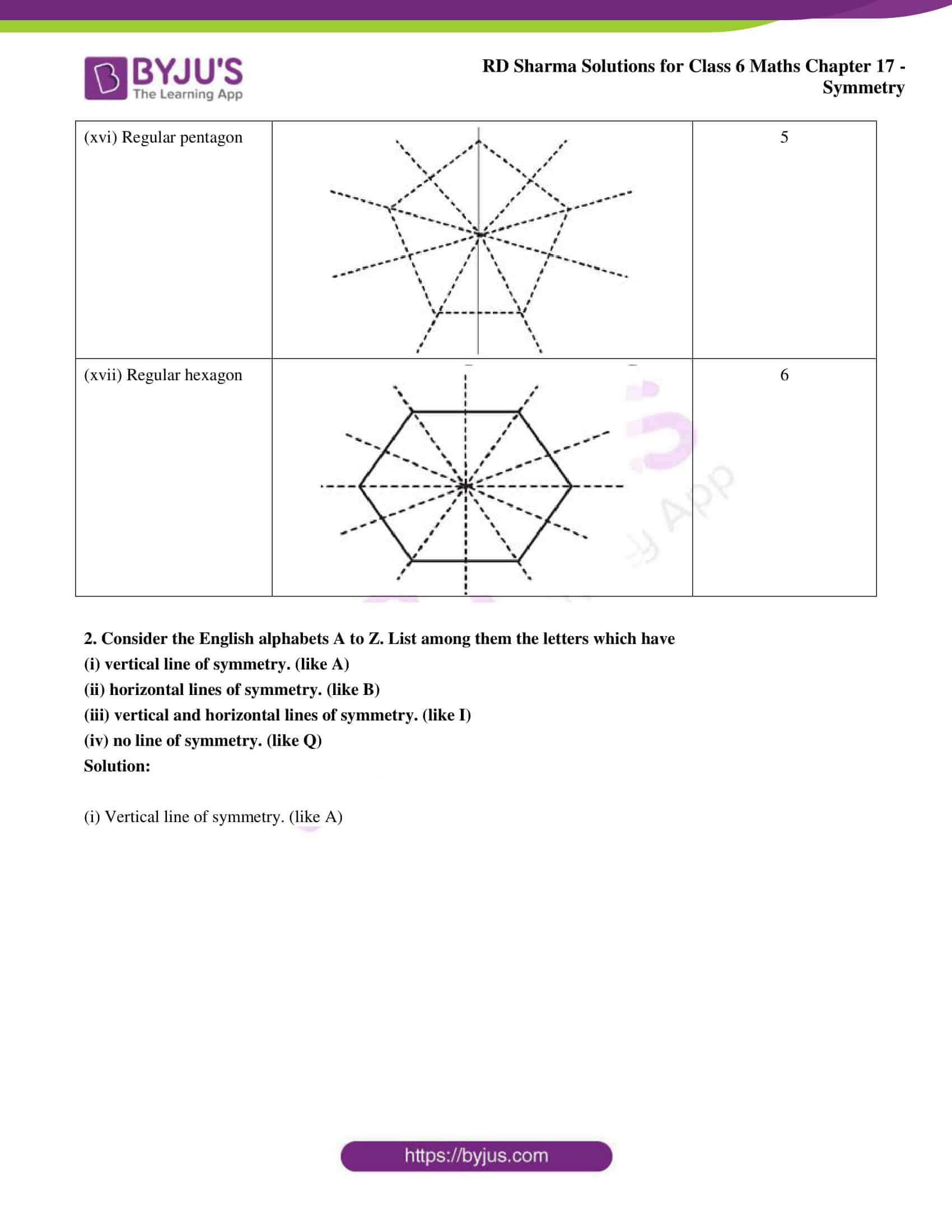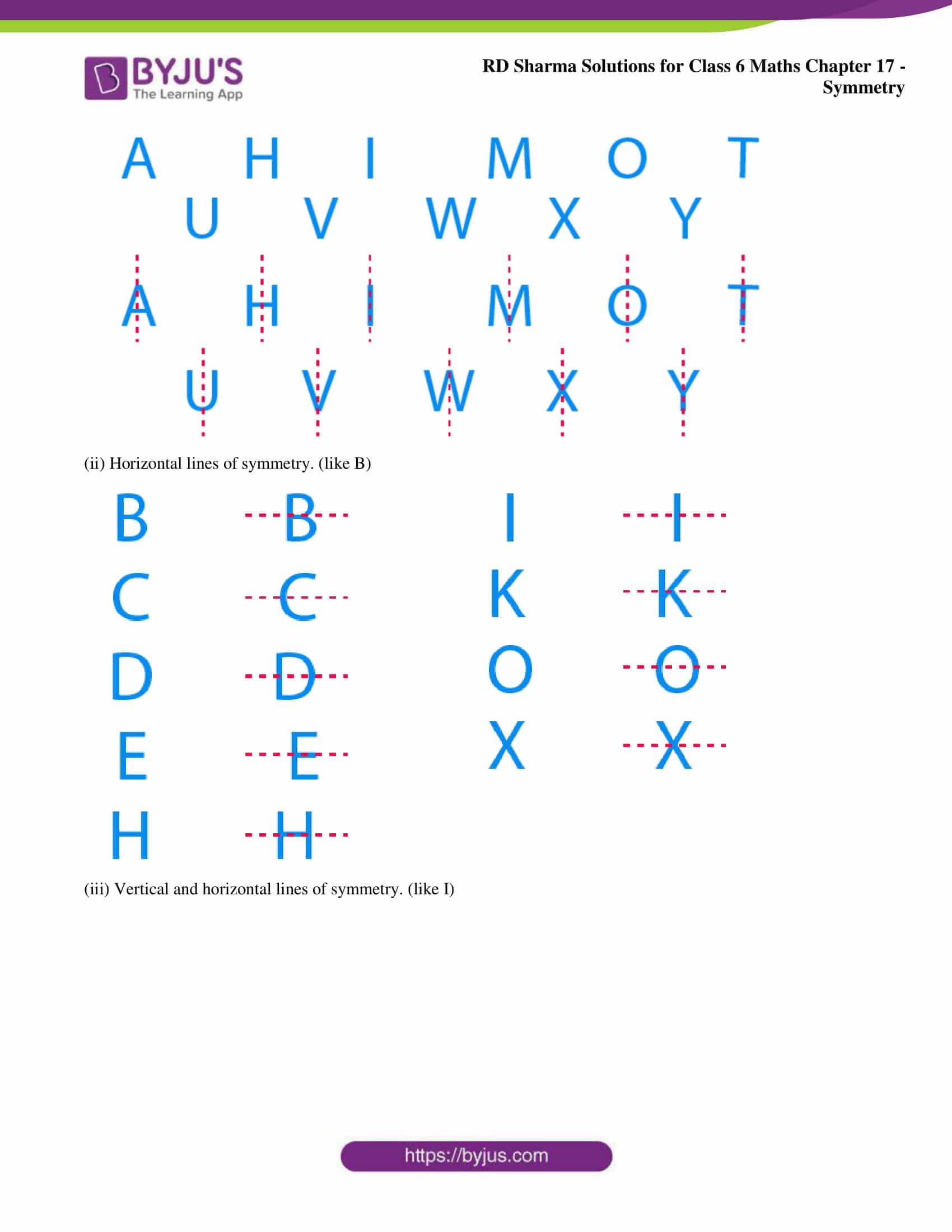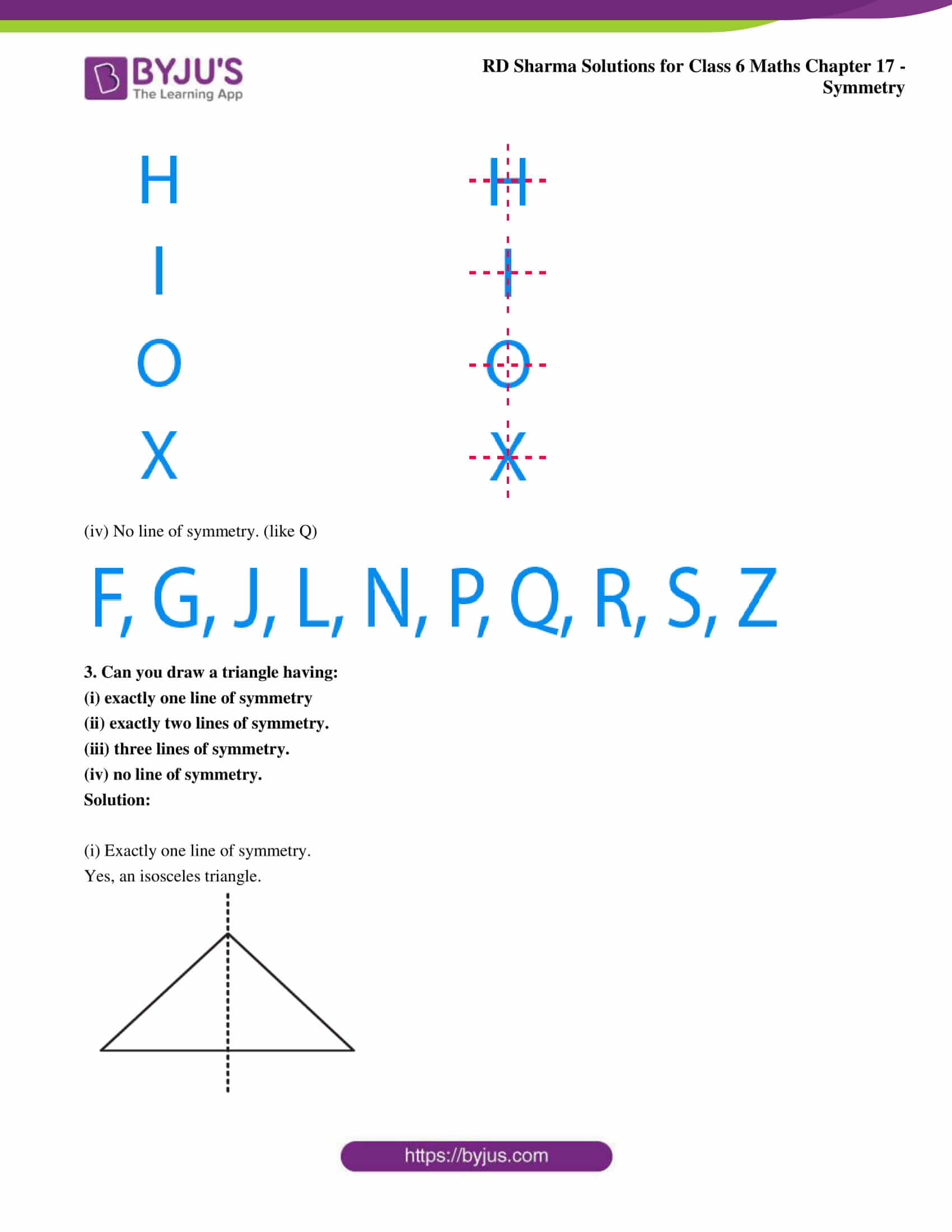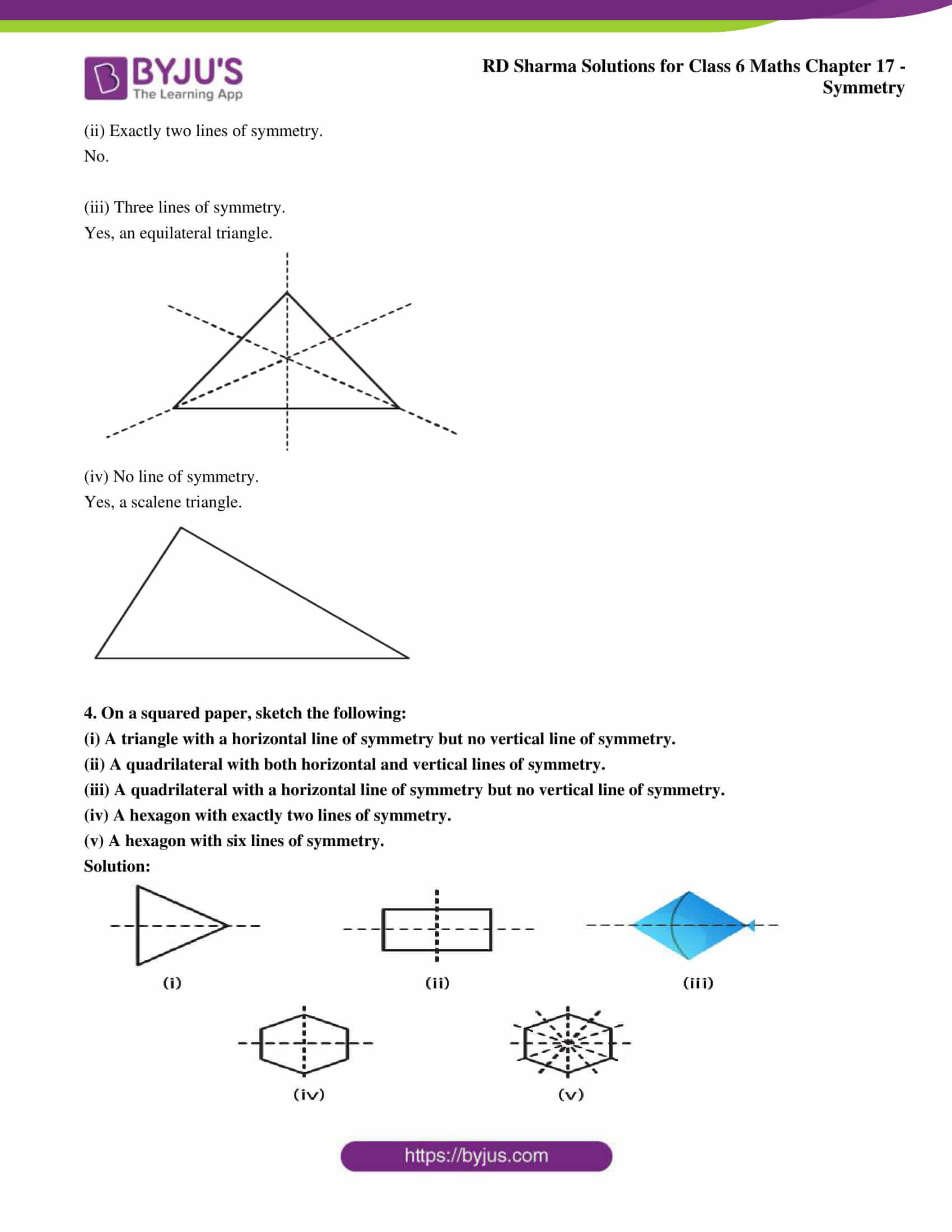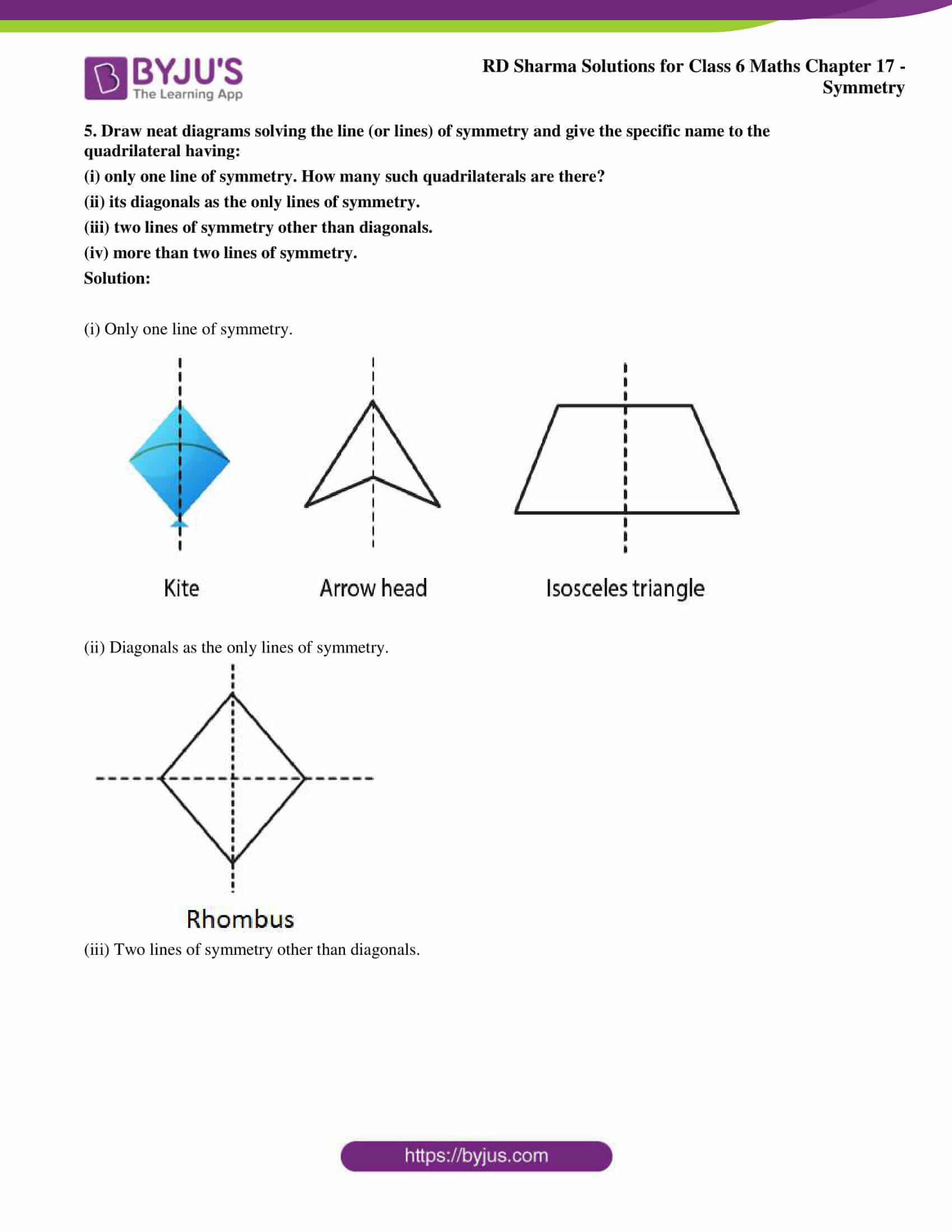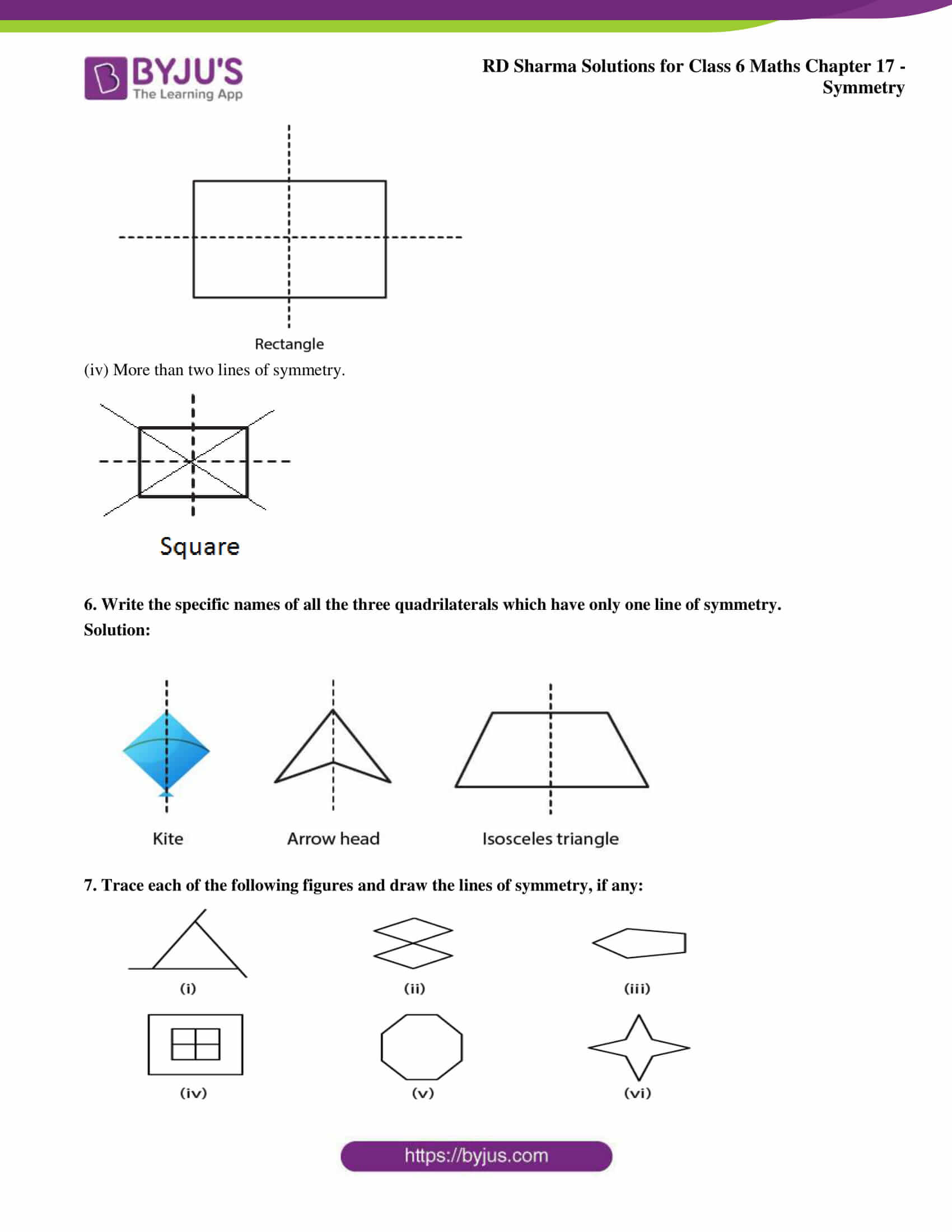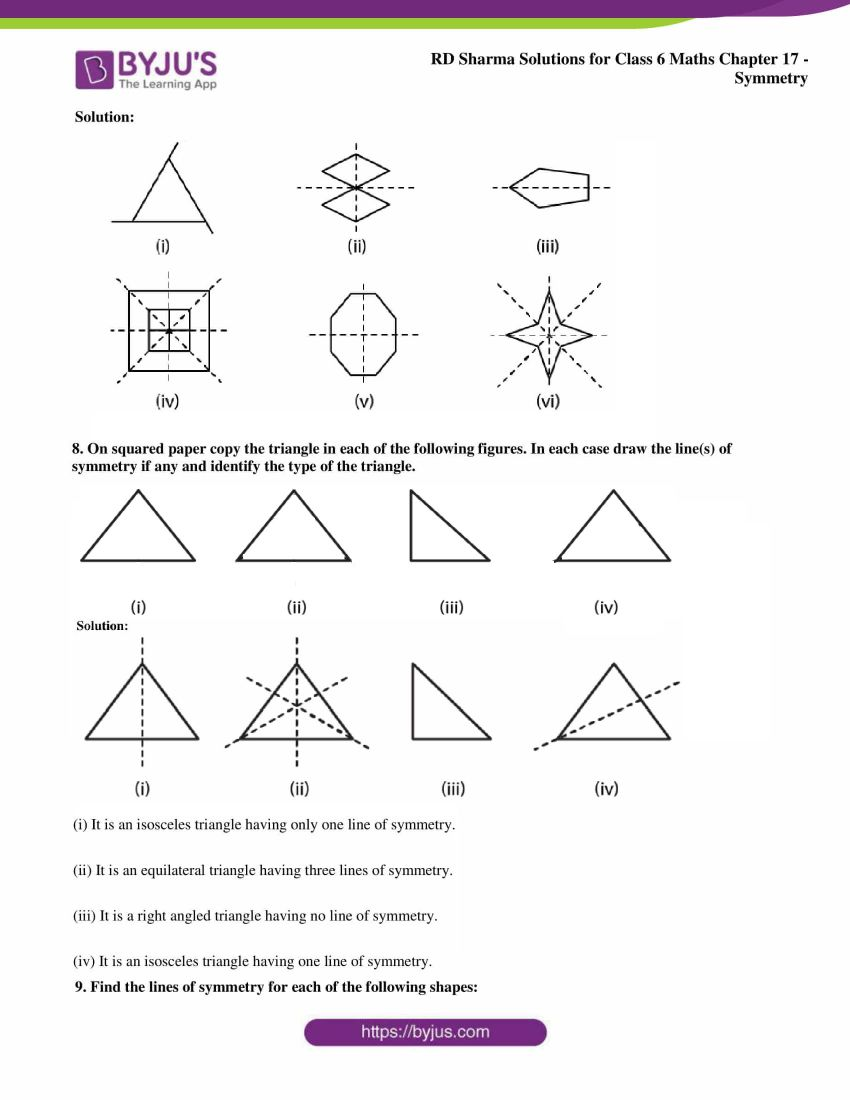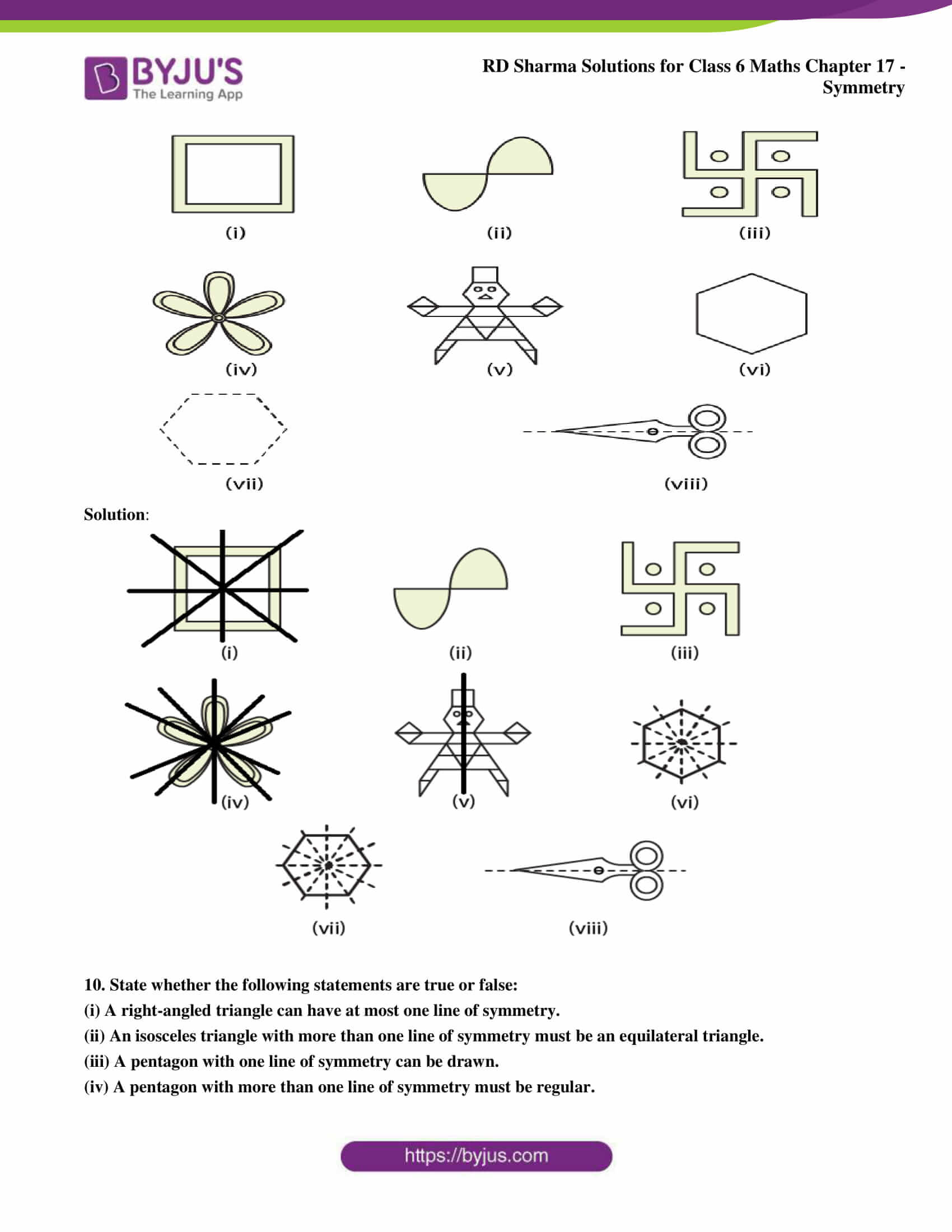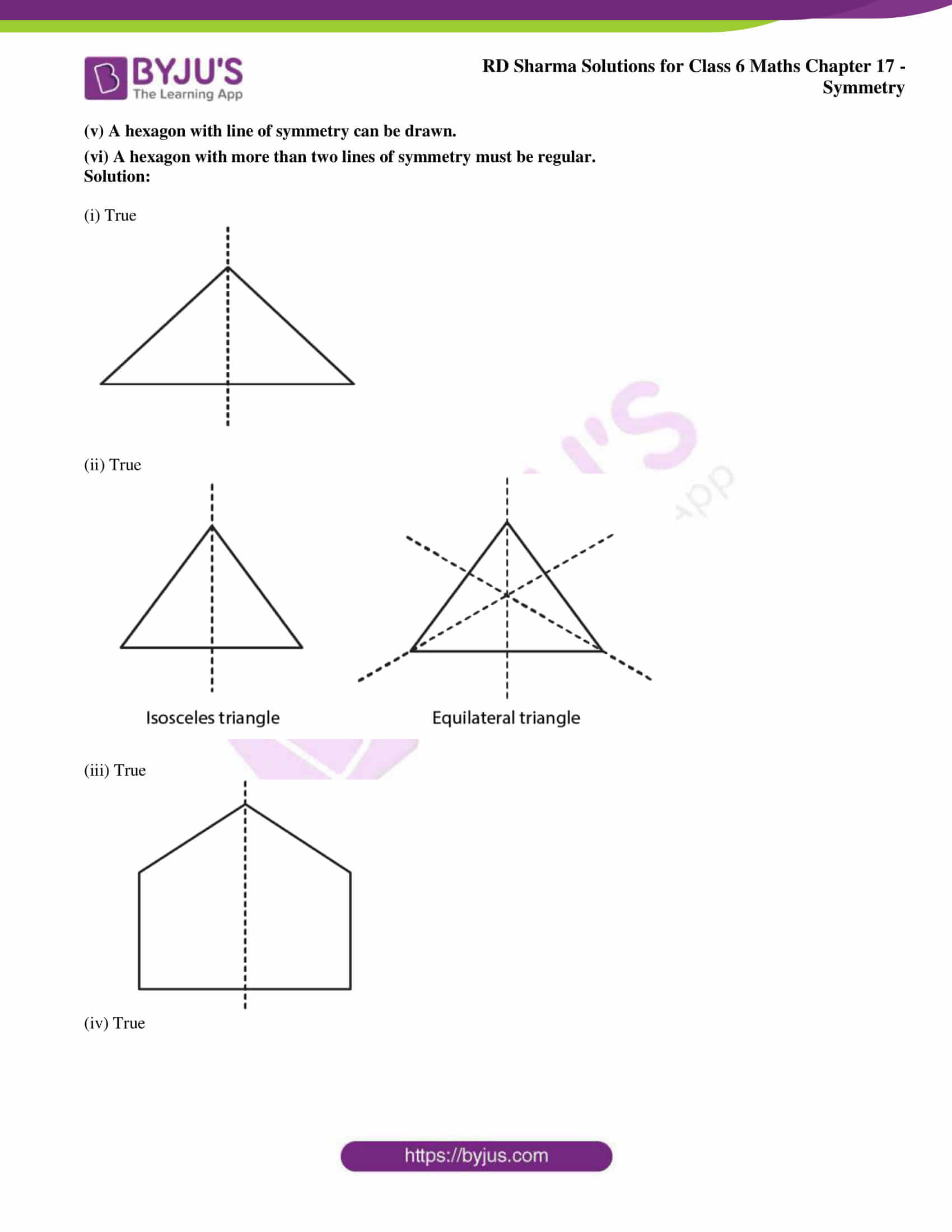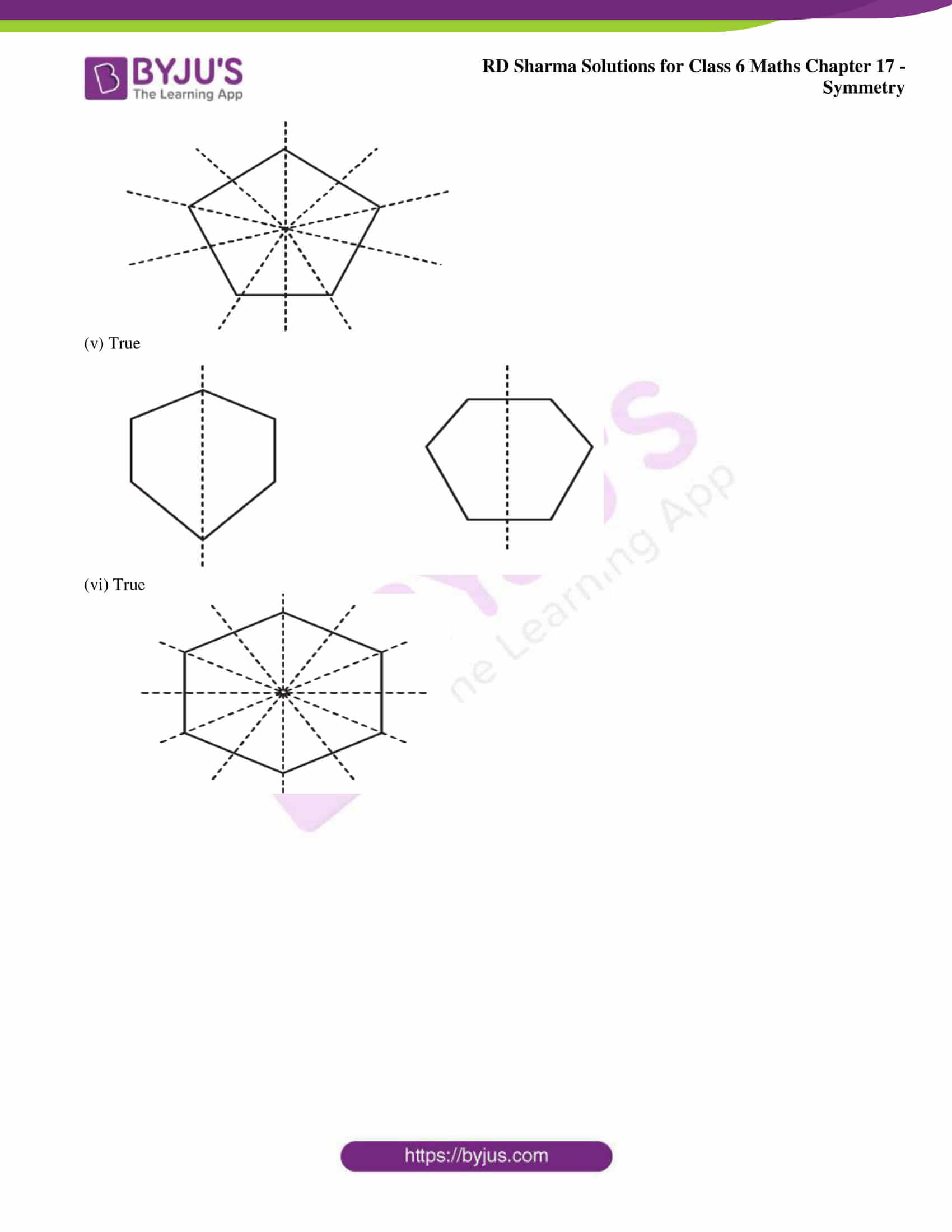### Exercise 17.3 page: 17.11

1. Complete the following table: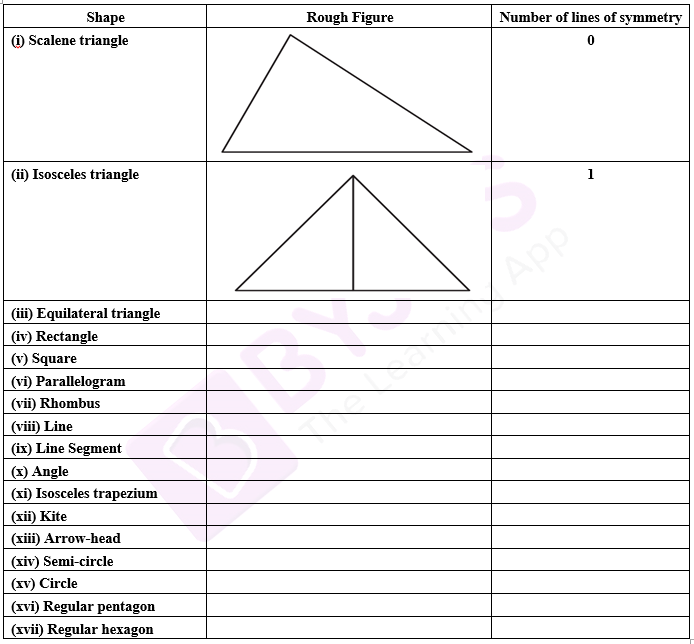Solution:

 Shape Rough Figure Number of lines of symmetry (i) Scalene triangle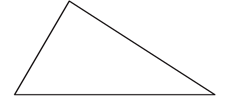0 (ii) Isosceles triangle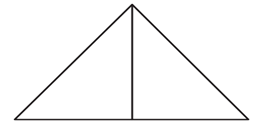1 (iii) Equilateral triangle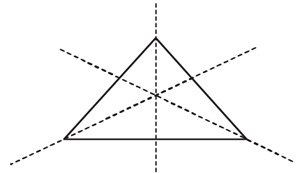3 (iv) Rectangle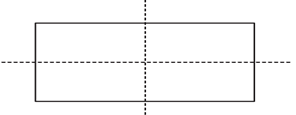2 (v) Square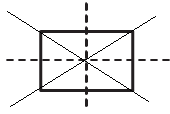4 (vi) Parallelogram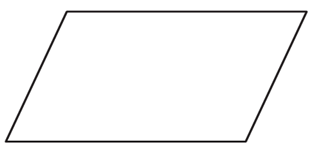0 (vii) Rhombus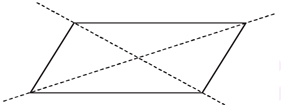2 (viii) Line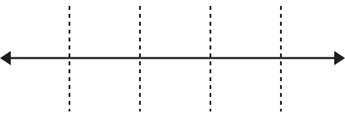Infinitely many (ix) Line Segment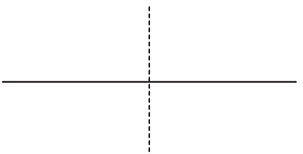1 (x) Angle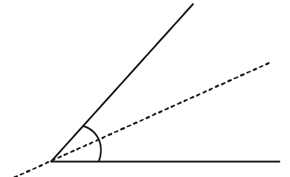1 (xi) Isosceles trapezium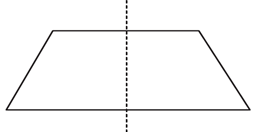1 (xii) Kite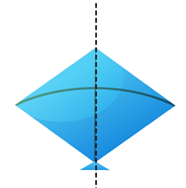1 (xiii) Arrow-head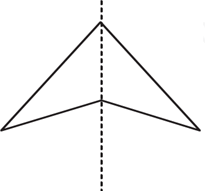1 (xiv) Semi-circle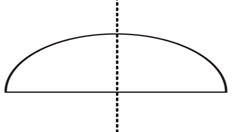1 (xv) Circle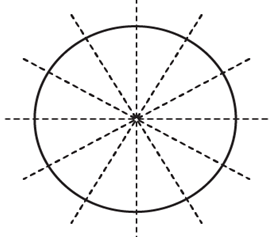Infinitely many (xvi) Regular pentagon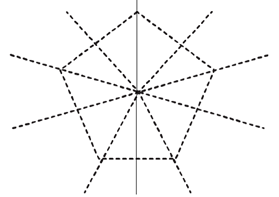5 (xvii) Regular hexagon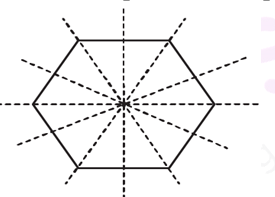6

2. Consider the English alphabets A to Z. List among them the letters which have

(i) vertical line of symmetry. (like A)

(ii) horizontal lines of symmetry. (like B)

(iii) vertical and horizontal lines of symmetry. (like I)

(iv) no line of symmetry. (like Q)

Solution:

(i) Vertical line of symmetry. (like A)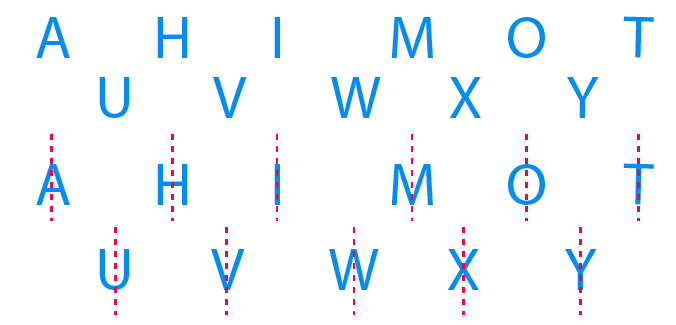(ii) Horizontal lines of symmetry. (like B)(iii) Vertical and horizontal lines of symmetry. (like I)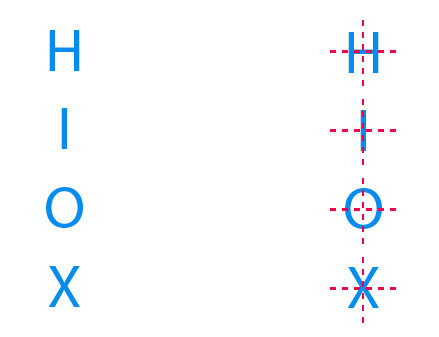(iv) No line of symmetry. (like Q)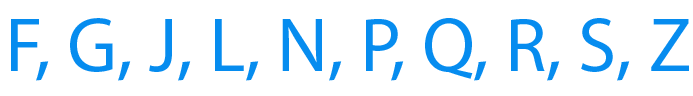3. Can you draw a triangle having:

(i) exactly one line of symmetry

(ii) exactly two lines of symmetry.

(iii) three lines of symmetry.

(iv) no line of symmetry.

Solution:

(i) Exactly one line of symmetry.

Yes, an isosceles triangle.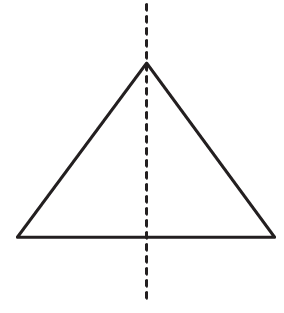(ii) Exactly two lines of symmetry.

No.

(iii) Three lines of symmetry.

Yes, an equilateral triangle.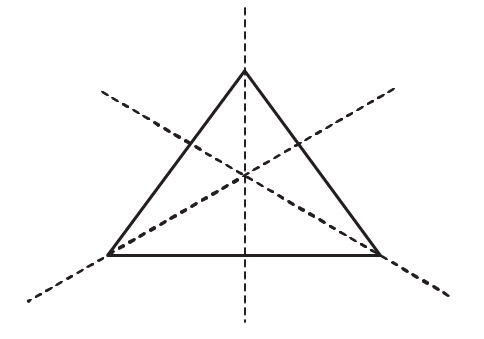(iv) No line of symmetry.

Yes, a scalene triangle.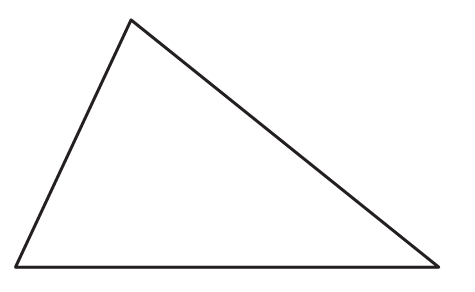4. On a squared paper, sketch the following:

(i) A triangle with a horizontal line of symmetry but no vertical line of symmetry.

(ii) A quadrilateral with both horizontal and vertical lines of symmetry.

(iii) A quadrilateral with a horizontal line of symmetry but no vertical line of symmetry.

(iv) A hexagon with exactly two lines of symmetry.

(v) A hexagon with six lines of symmetry.

Solution: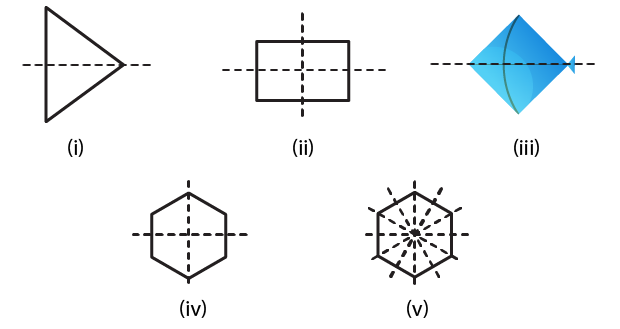5. Draw neat diagrams solving the line (or lines) of symmetry and give the specific name to the quadrilateral having:

(i) only one line of symmetry. How many such quadrilaterals are there?

(ii) its diagonals as the only lines of symmetry.

(iii) two lines of symmetry other than diagonals.

(iv) more than two lines of symmetry.

Solution:

(i) Only one line of symmetry.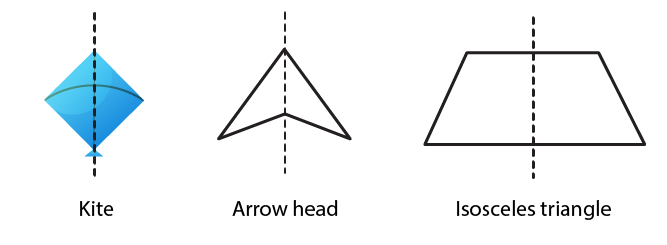(ii) Diagonals as the only lines of symmetry.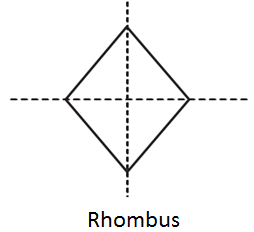(iii) Two lines of symmetry other than diagonals.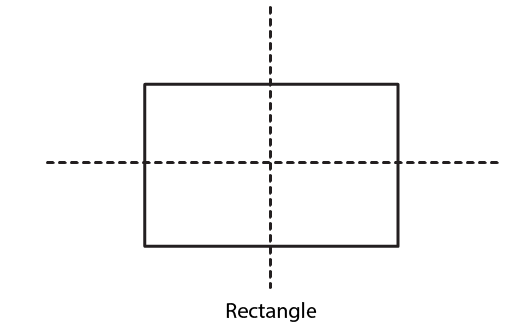(iv) More than two lines of symmetry.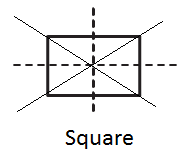6. Write the specific names of all the three quadrilaterals which have only one line of symmetry.

Solution:7. Trace each of the following figures and draw the lines of symmetry, if any: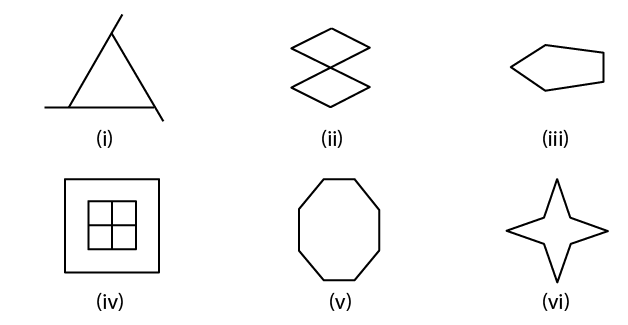Solution: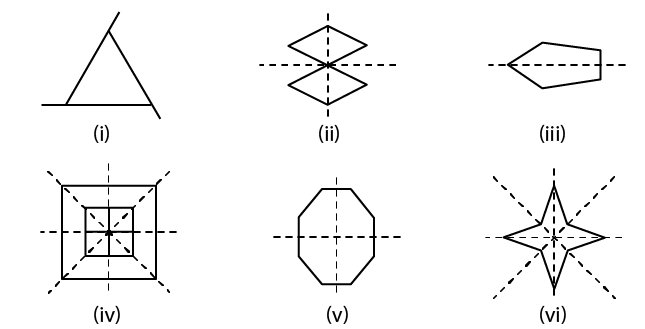8. On squared paper copy the triangle in each of the following figures. In each case draw the line(s) of symmetry if any and identify the type of the triangle.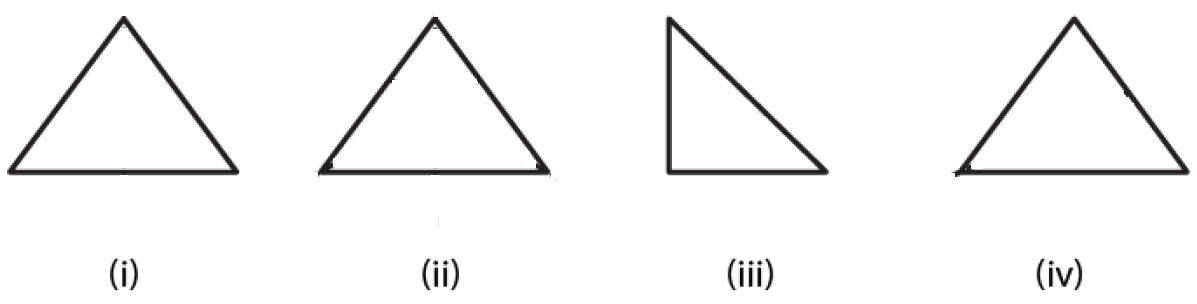Solution: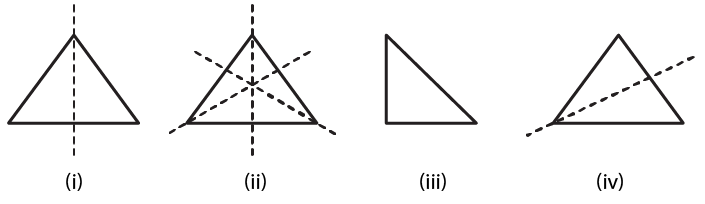(i) It is an isosceles triangle having only one line of symmetry.

(ii) It is an equilateral triangle having three lines of symmetry.

(iii) It is a right angled triangle having no line of symmetry.

(iv) It is an isosceles triangle having one line of symmetry.

9. Find the lines of symmetry for each of the following shapes: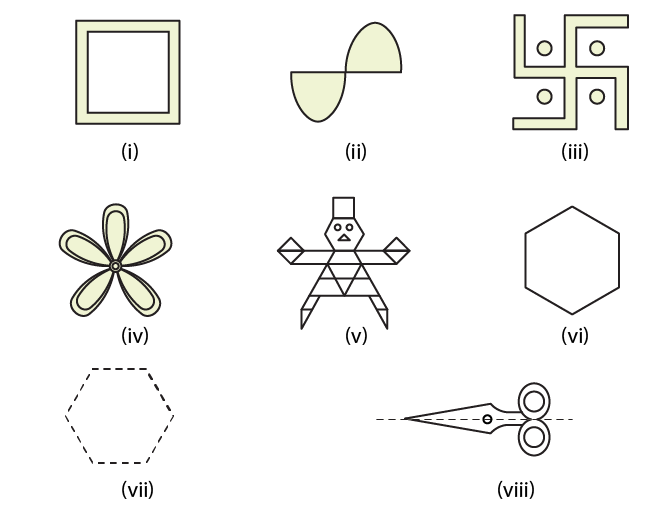Solution: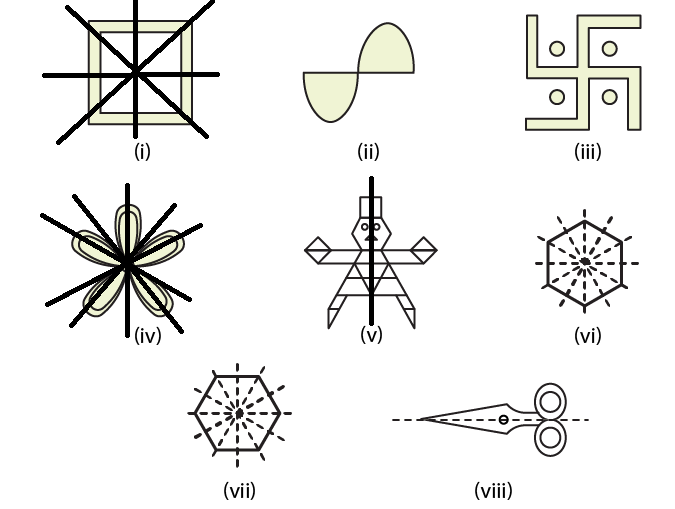10. State whether the following statements are true or false:

(i) A right-angled triangle can have at most one line of symmetry.

(ii) An isosceles triangle with more than one line of symmetry must be an equilateral triangle.

(iii) A pentagon with one line of symmetry can be drawn.

(iv) A pentagon with more than one line of symmetry must be regular.

(v) A hexagon with line of symmetry can be drawn.

(vi) A hexagon with more than two lines of symmetry must be regular.

Solution:

(i) True(ii) True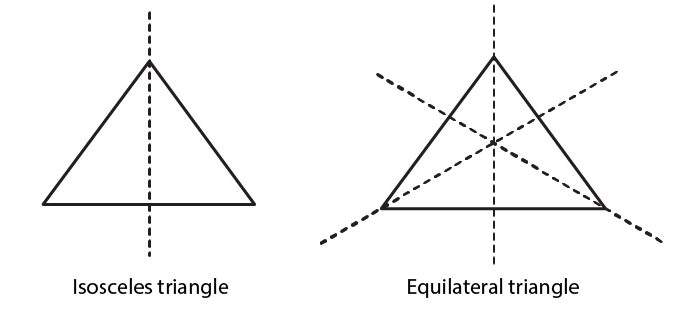(iii) True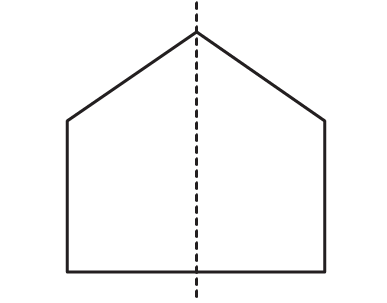(iv) True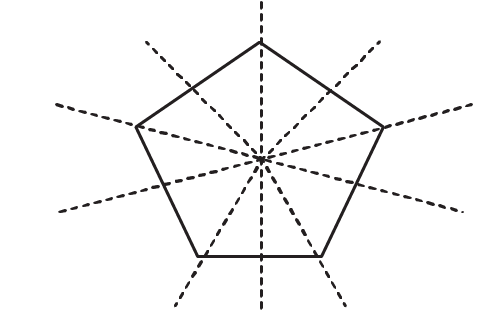(v) True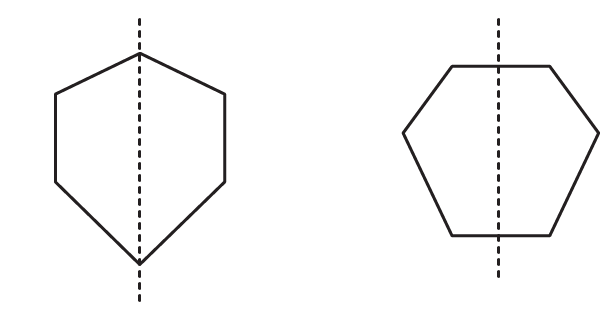(vi) True